## Introduction

Periodically driven disordered granular media and colloidal suspensions display an intriguing transition from reversible to irreversible deformation as the amplitude of the loading overcomes a threshold value1,2,3,4,5,6,7,8. A very similar behavior is also observed in simulations of amorphous solids under cyclic shear9,10,11, suggesting that it might be a general feature of slowly relaxing disordered systems. These results are interesting not only because they help to clarify how irreversible behavior arise from reversible microscopic dynamics1 but also because they shed light on the nature of yielding in amorphous media12. In the light of these results, we can interpret the onset of plasticity as a phase transition from a reversible elastic dynamics to an irreversible plastic deformation. The relation between yielding in crystals, where irreversibility is associated to the motion of defects, and glasses is still a subject of current investigation.

Colloidal suspensions are becoming a widely used tool to study experimentally the properties of matter since the change of scale from the atomistic to the colloidal ones allows to directly observe phenomena that would otherwise not be within experimental reach. Depending on the experimental conditions colloidal particles can be assembled into desired states, from perfectly ordered crystals13 to disordered amorphous glasses14. In between these two extremes lie colloidal polycrystals, where ordered crystalline regions are separated by extended grain boundaries formed by dislocation arrays15,16,17,18. The application of a cyclic shear to colloidal polycrystals allows to follow at the same time the dynamics of individual particles (“atoms”) and the large-scale response of the polycrystalline texture15,16,17,18.

Here, we investigate the response of colloidal polycrystals to cyclic loading by molecular dynamics simulations and explore the coupling between dislocations, grain boundaries and the dynamics of individual colloidal particles. The system we consider is similar to the experimental setup studied in ref. 18, where a colloidal crystal is doped with larger colloidal particles whose concentration is used to control the grain size. Our simulations show that for large enough strain amplitudes grain boundaries eventually disappear and the system orders, while at small amplitude the system settles after an initial transient to a state very close to the original metastable configuration and reversibility is maintained. Thus, there is an effective phase transition controlled by the strain amplitude between asymptotic steady-states. The dynamics of this transition is found to be of a transient character, and leads to the presence of crackling noise in the system response. We also show that higher impurity concentration leads to a larger yield strain. The depinning of grain boundaries or cross-over to irreversibility is a non-equilibrium version of the Zener pinning in grain boundary coarsening in metals in the presence of impurities19,20.

## Results and Discussion

In 2D triangular colloidal crystals, five coordinated particle, known as positively charged disclinations (+1), and seven coordinated particles, corresponding to negatively charged disclinations (−1), exist as defects. The likelihood of an individual disclination occurring is low. However, the combination of five and seven coordinated particles with a small additional energy creates edge dislocations. A regular array of dislocations then creates a grain boundary separating differently oriented crystals, as shown in Fig. 1. The top panel in Fig. 1 corresponds to the local crystal orientation, θ, and the bottom panel represents the nearest neighbour characteristics, Ncoord, of each particle. A careful study shows that arrays of dislocations are mainly arranged along the grain boundaries with high misorientations, which is consistent with Frank condition, n sin , where n is the line density along the grain boundary with the corresponding misorientaion 21,22.Figure 1: Grain orientations and boundaries before and after the applied strain.

Once the polycrystalline samples are prepared, they undergo cyclic deformation with different values of strain amplitude γ0. For low values of γ0, the grain boundary network recovers its initial configuration after each cycle (see Fig. 1 second column and Video S1 in Supplementary information), whereas for higher values of γ0 all dislocations annihilate from the initial polycrystalline samples (see Fig. 1 last column and Video S3 in Supplementary information). For intermediate values of γ0, however, a memory of the initial grain boundary structure can still be observed after cycling (see Fig. 1 third column and Video S2 in Supplementary information). To quantify the response to cyclic shear, we measure the time evaluation of the disclination density ρDis, defined as the number density of particles having either 5 (+1 disclination) or 7 (−1 disclination) neighbours, for different values of γ0 as shown in Fig. 2(a). For low values of γ0, ρDis remains unchanged which is consistent with the previous discussions. However, when γ0 > 0.044, ρdis rapidly decreases and reaches a steady state value. When the impurity concentration increases, a large value of strain amplitude is required to reach the asymptotic disclination density, which is shown in Fig. 2(b). It could be understood as follows: from Fig. 2(b), disclination density increases by 66.7% when impurity density is increased by 200% that explains the increase of impurity density along the grain boundaries. Segregation of impurities in the grain boundaries leads to lowering of total free energy23,24 and therefore the increase of yield stress is observed. The asymptotic disclination density ρDis corresponds to the disclinations created by the presence of larger particles and can be estimated as l, where c ≈ 2.5 and ρl is the density of the larger particles. We notice that the final disclination density after 600 cycles depends on the sample size and the transition becomes sharper when L is larger. See Fig. 2(c).

In order to understand the behavior of the dynamics at the particle level and the role of reversibility and irreversibility, we calculate the mean square displacements (MSDs), Δr2, with respect to the initial configurations averaged over 100 samples. Figure 3(a) shows that for small values of γ0, Δr2 saturates after initial transient, whereas for higher values of γ0, the particle displacements become diffusive. That is, for large enough values of γ0, MSD increases in a linear way, implying irreversible single particle behavior. When the system comes to the related steady state, one can extract the diffusivity,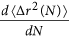, which is plotted in Fig. 3(b) for different system sizes. When γ0 0.044, the system remains in a quiescent state, however for larger values of γ0, diffusivity increases sharply. In short, both the quantities indicate that the colloidal polycrystals respond differently depending on the amplitude of γ0.

In order to measure the dynamical heterogeneity in the system, we compute the probability distribution function (PDF) of the particle displacement magnitude (D) after N = 600 cycles with respect to the initial configurations for different values of γ0 (see Fig. 3(c)). Histograms for different values of γ0 are collapsed by rescaling D with average displacement 〈D〉. The first part of the collapsed data in Fig. 3(d) scales as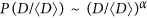and α = 0.96, i. e. the exponent is very close to unity. The tail part exhibits an exponential decay when γ0 > 0.0625 and for smaller values decays very rapidly. It is interesting to note that both for small and big γ0-amplitudes the histograms are in any case quite similar and that Fig. 3(c) connects the reversibility-irreversibility change to the largest typical values of D found: when these start to be of the order of unity, reversibility is lost. This is seen also by looking at the Inset of Fig. 3(d). The single-particle behavior averaged and measured by 〈D〉 shows no direct signs of collective behavior, but as the displacement magnitude grows - and reversibility is destroyed - collective effects and restructuring starts.

In order to understand the plastic deformation at the particle level, we compute the local shear strain,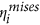, for each atom at the end of each cycle25. To this end, we consider the initial configurations as references and find the local transformation matrix Ji that best maps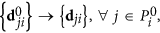where d’s are vector separations (row vectors) between atom j and i (superscript 0 means the reference configuration). Here, j is one of atom i’s nearest neighbours, and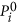is the total number of nearest neighbours of atom i, at the reference configurations. Ji is determined by minimising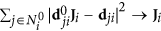. For each Ji, the local Lagrangian strain matrix is computed as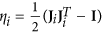. Then local shear invariant is calculated for each atom i as follows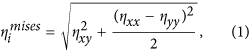and average is taken over all atoms present in the system.

First, we have plotted 〈ηmises〉 versus N as shown in Fig. 4(a) which has qualitatively similar behavior as 2(a). The strain shows large, transient fluctuations close to the transition, as plotted in Fig. 5(a) where we plot the strain rate (dηmises〉)/(dN), denoted as fmises, as a function of number of cycles for different values of γ0. Note how the strain signal evolves with N for the two larger amplitudes. This crackling noise is the signature of the non-equilibrium transition of grain boundary depinning which can be clearly seen in Videos S2 and S3 in Supplementary Information. We then compute the strain rate distribution and find that it exhibits a scaling as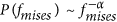with α = 1.17 for γ0 = 0.063 (see Fig. 4(b)). However, with decreasing the strain amplitude towards γ0 = 0.031, the exponent α increases. This is because for lower values of γ0, large events are less likely as shown in fmises(N) vs N plot. To quantify the energy dissipation during the plastic events, hysteresis loop area is computed from the shear stress against applied strain as shown in Fig. 4(c) and plotted as a function of strain amplitude (see Fig. 4(d)) where transition is attributed by observed energy dissipation. In a similar study Laurson et al.26 has characterized two phases corresponding to the jammed and the moving state by computing hysteresis loop area when the crystalline solids with dislocations are stressed cyclically. It has been explained in the light of collective dislocation dynamics.

To quantify the statistics of crackling noise in colloidal crystal, we compute the distribution of avalanche sizes which is defined as the sequence of values of fmises(N) exceeding some threshold value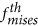to subtract an uncorrelated back ground. If an avalanche starts at N = 0 and ends at N = n, the size of an avalanche of duration n is defined as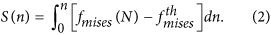Distributions of avalanche sizes are reported in Fig. 5(b). As expected in general for crackling noise, the distribution crucially depends on the strain amplitude. For higher values of γ0, the avalanche distribution decays as a power law, P(S) ~ Sτ, where τ = 1.57 and the exponent τ increases as we move towards the lower values of strain amplitudes. The clearest power law is observed at the critical point γ0 = 0.063 where the cutoff increases with the system size L as shown in Fig. 6. These distributions result from an integration of the bursty dynamics (Fig. 5) which is of transient character. Eventually after an amplitude-dependent number of cycles the noise develops a stationary character. In passing notes, we also observe sliding of dislocations and rotation of grains which induce the plastic deformations in polycrystalline materials as described in ref. 27. See Fig. 7.

## Summary

In conclusion, we have simulated a colloidal polycrystal under cyclic shear and observed a transition from reversible deformation in which the grain structure is preserved after each cycle and an irreversible phase leading to crystal ordering and the disappearance of grain boundaries and dislocations. The transition displays all the typical signs of other non-equilibrium phase transitions such as finite size effects and power law distributed crackling noise. Our results indicate that colloidal polycrystals under oscillatory shear behave similarly as disordered particle assemblies and colloidal glasses, but the relevant degrees of freedom are here the topological defects instead of the particles themselves. The behavior we uncover has direct application to Zener pinning where inclusions are used to stop grain growth in a polycrystal19. The critical strain amplitude for irreversibility should correspond to the depinning stress for grain boundary growth20. Our results should be directly testable in colloidal particle experiments15,16,17,18.

## Methods

We consider a two-dimensional system with two types of particles, large (l) and small (s) interacting via a pair-wise Lennard-Jones potential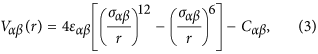where r is the distance between a particle of type α and one of type β(α, β = l, s). The functions Vαβ(r) = 0, when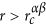a cutoff distance, which is defined by 3.0σαβ and the constant Cαβ ensures the continuity of Vαβ at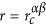. The parameters of the potential are chosen as follows: σ ≡ σs = 1.0, σl = 1.4σ and σls = 1.2σ are the particle diameters and εεll = 1.0, εss = 0.5ε, εls = 1.5ε. rc = 3.0σ are the energy parameters. In what follows, the lengths are normalized by σ. The typical numbers of the particles used in the system are Nl = 50 and Ns = 10000, with m = 1 for both with 100 samples for ensemble averaging over the initial conditions. The typical length of the 2D simulation box is L = 100. The unit of time is set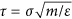. The equations of motion are solved numerically using the time-reversible measure-preserving Verlet and rRESPA integration scheme with a time step Δt = 0.005τ. To control the temperature the system is connected with Nose-Hoover thermostat and Lees-Edward boundary conditions are used to apply shear.

A polycrystalline structure, as shown in the top left of Fig. 1 (color shows the orientation of the crystals), is prepared as follows: the system is heated at T = 2.0ε/kB for the duration of 255 τ. Temperature is decreased to 0.01 ε/kB by a span of 150τ and the system is equilibrated for a time 5 × 103τ. Then, the system undergoes cyclic deformation along the x-direction with the following way: the system is sheared for 50 τ after which a similar reverse step is applied. The cycle is started and finished by a relaxation period of duration of 5 τ. A full cycle thus lasts 110 τ and the data presented below considers up to N = 600 cycles. To study the system size dependence, we consider systems of size L = 50 and L = 150 in addition to L = 100. To obtain a same strain amplitude for different sample sizes, the duration of shear phases are changed from 50 τ to 35.36 τ and 61.24 τ for L = 50 and L = 150, respectively. However, the relaxation time remains same for all the system sizes. Therefore, for L = 50 and L = 150, a full cycle consists of 80.72 τ and 132.48 τ, respectively. Molecular dynamics simulations using the above mentioned prescriptions are performed with LAMMPS (Large-scale Atomic/Molecular Massively Parallel Simulator: a free, open source Molecular Dynamics Simulators)28.